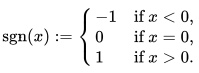# Sign Function (Signum): Definition, Examples

The sign function (or signum function) is a special function which returns:

• 1 for all x > 0 and
• -1 for all x < 0.

For x = 0, the value of the sign function is just zero.

It is a real-valued step function that tells us, numerically, whether a particular value of x is positive, negative, or zero.

## Sign Function: Definition

For every real number x, the sign function sgn(x) is defined as:Another definition of the signum function groups zero with the positive numbers. Under that definition,

• sgn(x) = 1 for x ≥ 0, and
• sgn(x) = -1.

## Special Properties

Any real number x can be written in terms of the signum function and the absolute value of the number.This means we can also write the signum function as## Derivative of the Sign Function

For any x not equal to zero, the derivative of x is equal to the sign function. The derivative of the sign function is just equal to zero, except at zero, where the derivative does not exist.

## Generalizing to Complex Numbers

The signum function doesn’t only work for real numbers; it can also be defined for complex numbers, but there it needs a broader definition. We define the signum function over the complex numbers (which also includes the reals) as:If z ≠ 0, and if z is equal to zero, we say.

A quick check should convince you that this definition is a reasonable generalization of what we’ve already defined over a smaller space.

There is another generalization that might be more intuitive to use, though it is not as much an extension of the signum function as an extension of the ideas behind the sign function. This is written csgn, and is defined aswhere Im(z) is the imaginary part of a complex number z, and Re(z) the real part.

## Signum Function in Software

Every major Computer Algebra System (CAS) has an equivalent of a sign function. For example:

Examples:

• SIGN function in Derive,
• Signum and piecewise functions in Maple V ,
• Sign[x] in Mathematica.

Note that each of the major CAS uses a slightly different definition. This is because different branches of mathematics define the function in slightly different way (Jeffrey et. al, n.d.).

## References

Calculus I Homework: Calculating Limits Using the Limit Laws Page 1. Retrieved July 13, 2021 from: http://cda.morris.umn.edu/~mcquarrb/teachingarchive/M1101/HW/2.3.pdf
Jeffrey et al. Integration of the signum, piecewise and related functions. Retrieved July 13, 2021 from: http://citeseerx.ist.psu.edu/viewdoc/download?doi=10.1.1.70.4127&rep=rep1&type=pdf

CITE THIS AS:
Stephanie Glen. "Sign Function (Signum): Definition, Examples" From StatisticsHowTo.com: Elementary Statistics for the rest of us! https://www.statisticshowto.com/sign-function/